Ex 11.1

Chapter 11 Class 10 Constructions
Serial order wise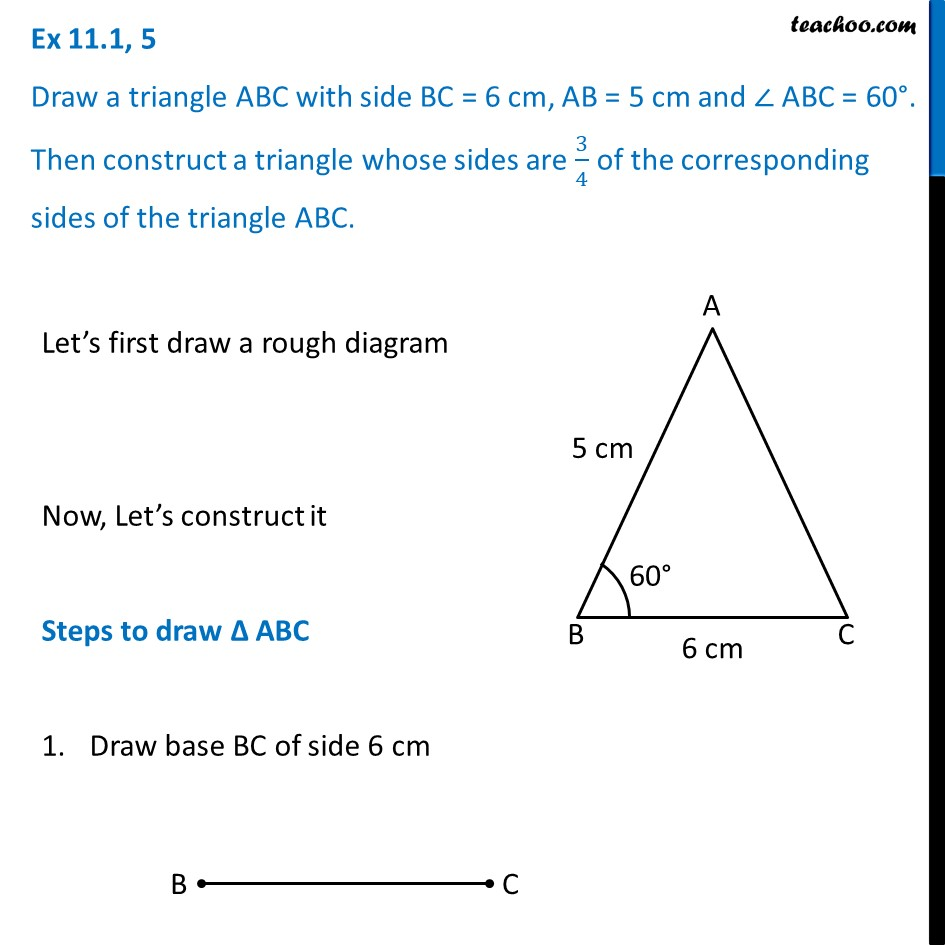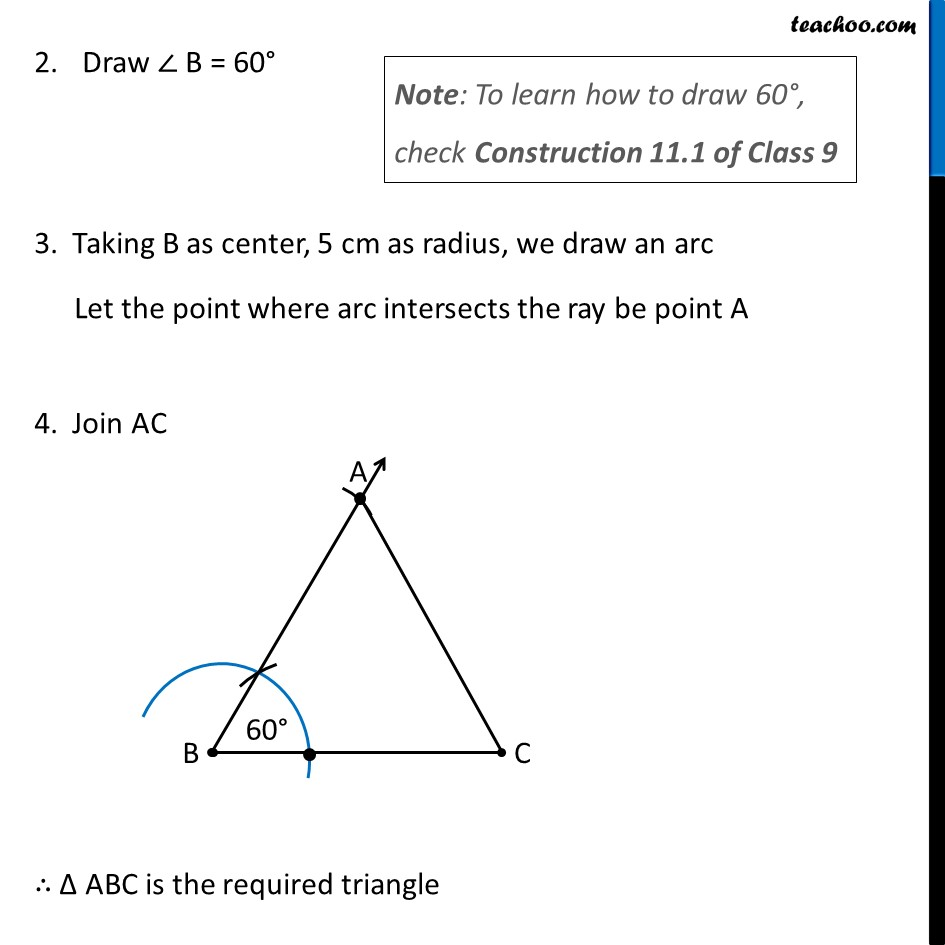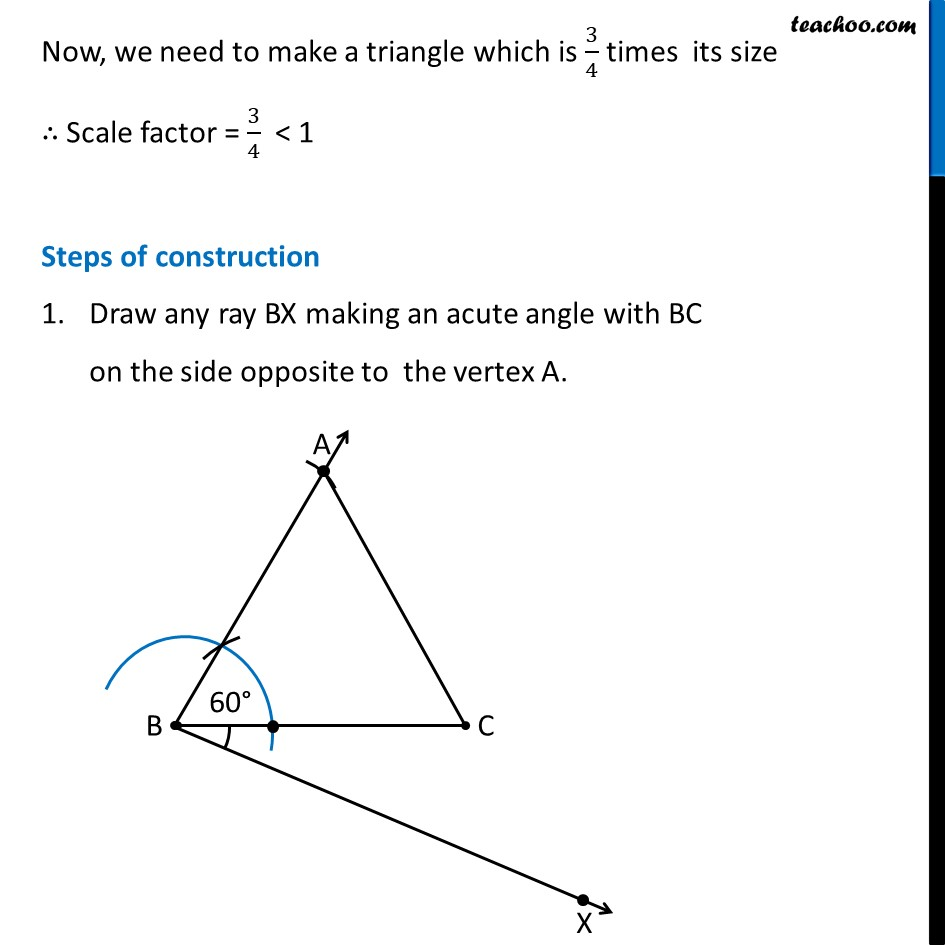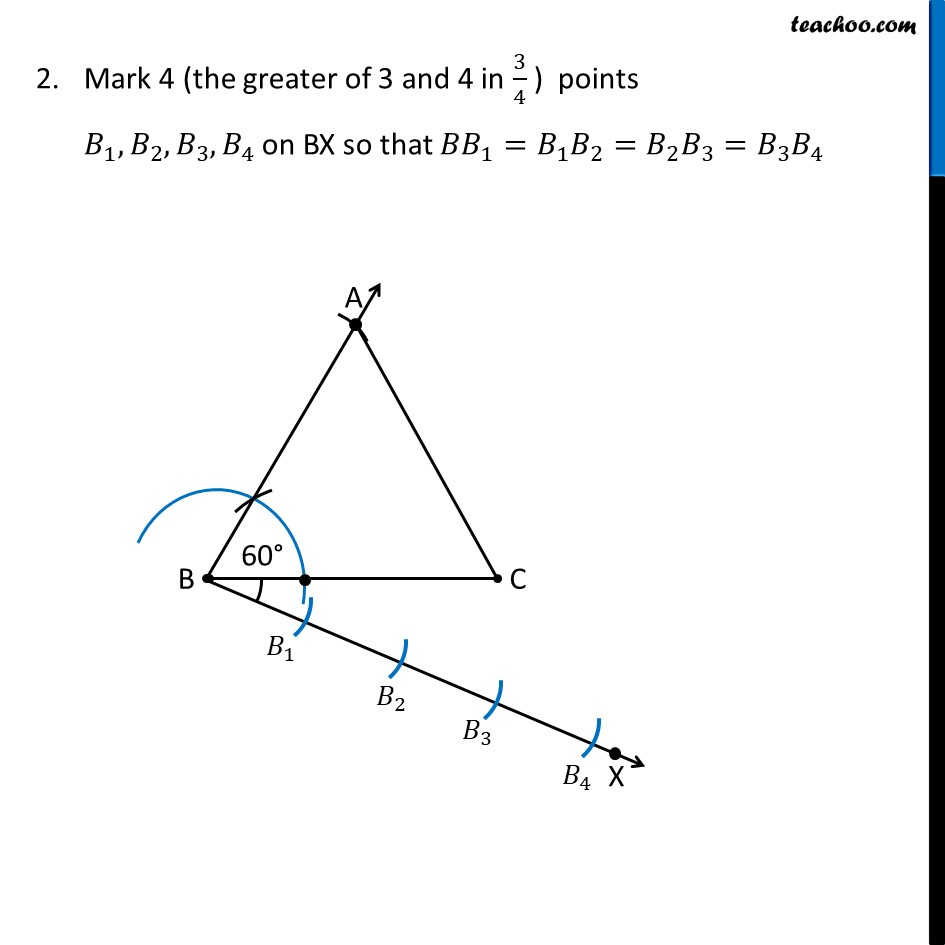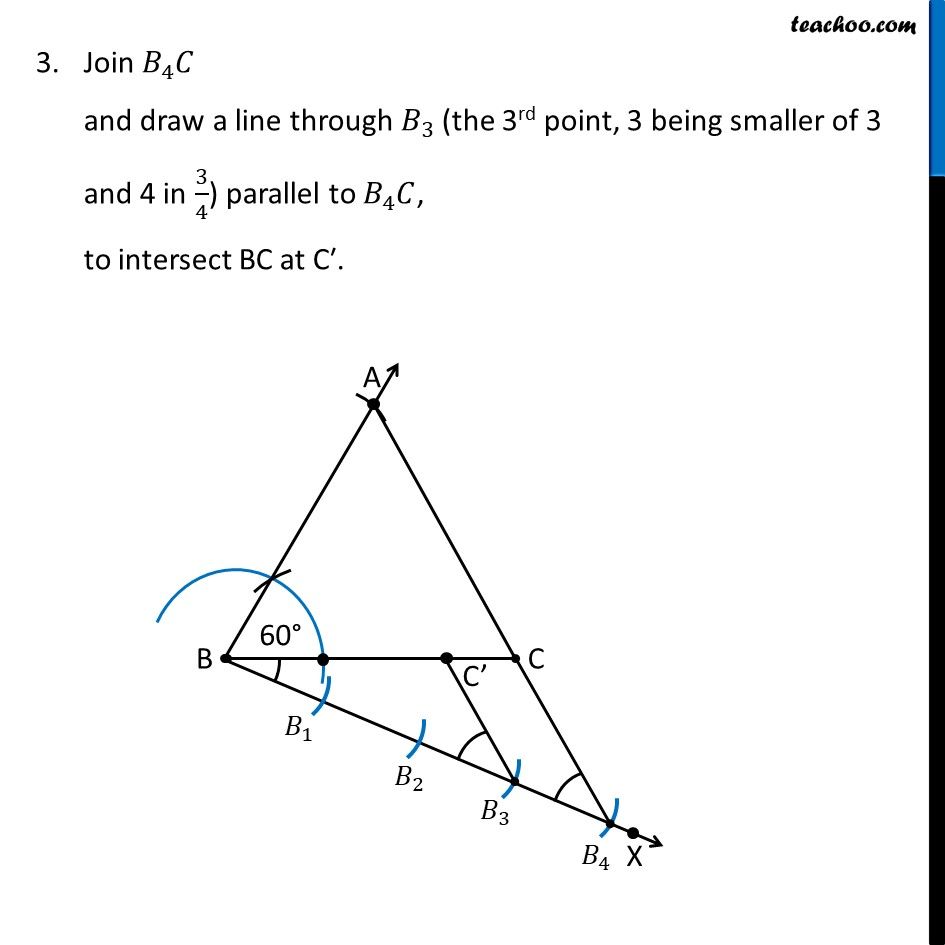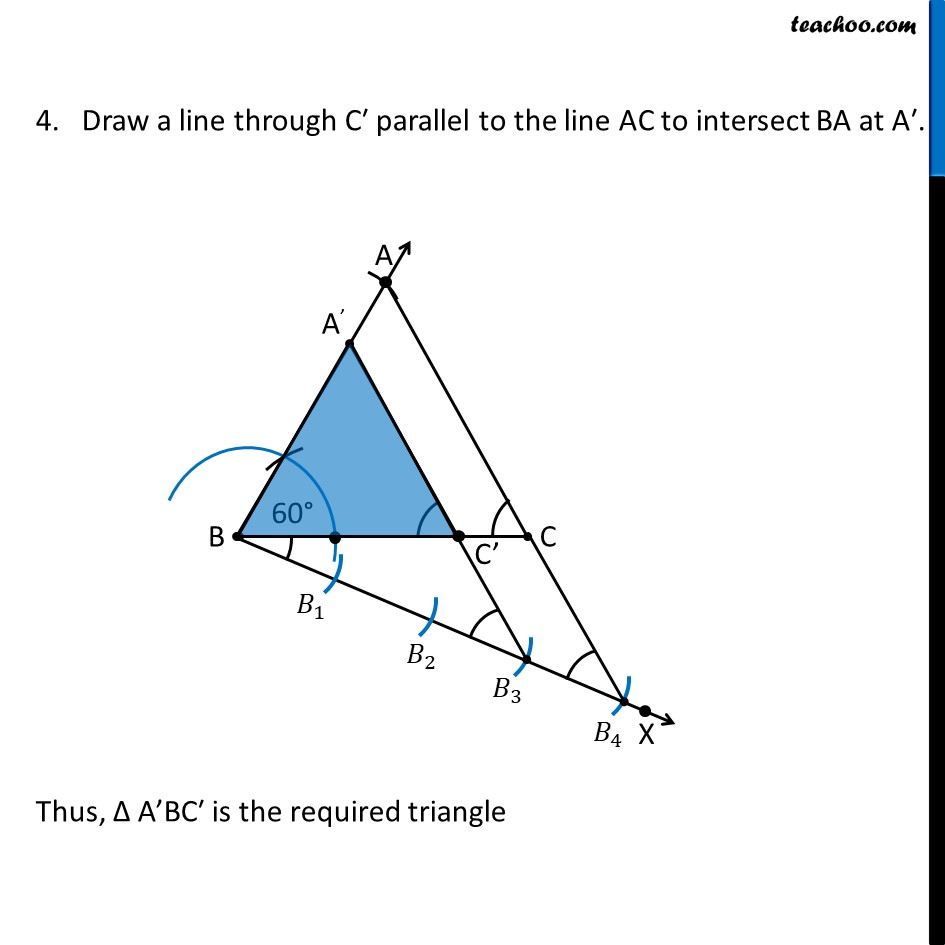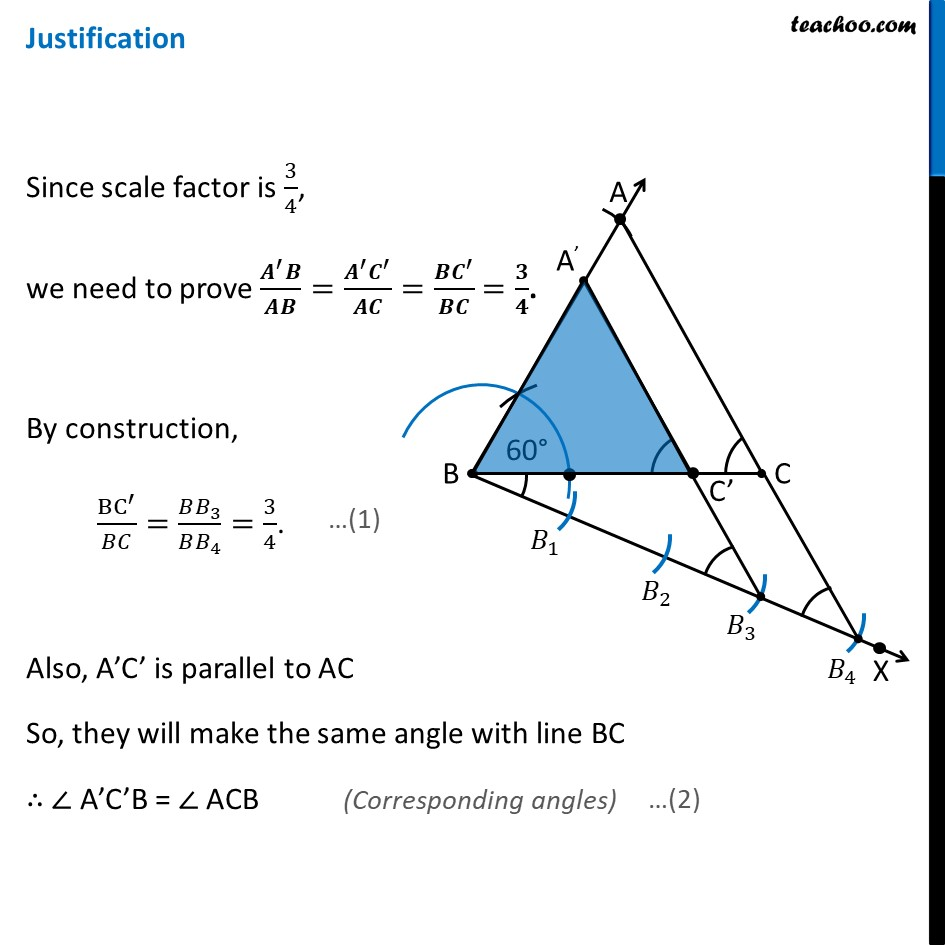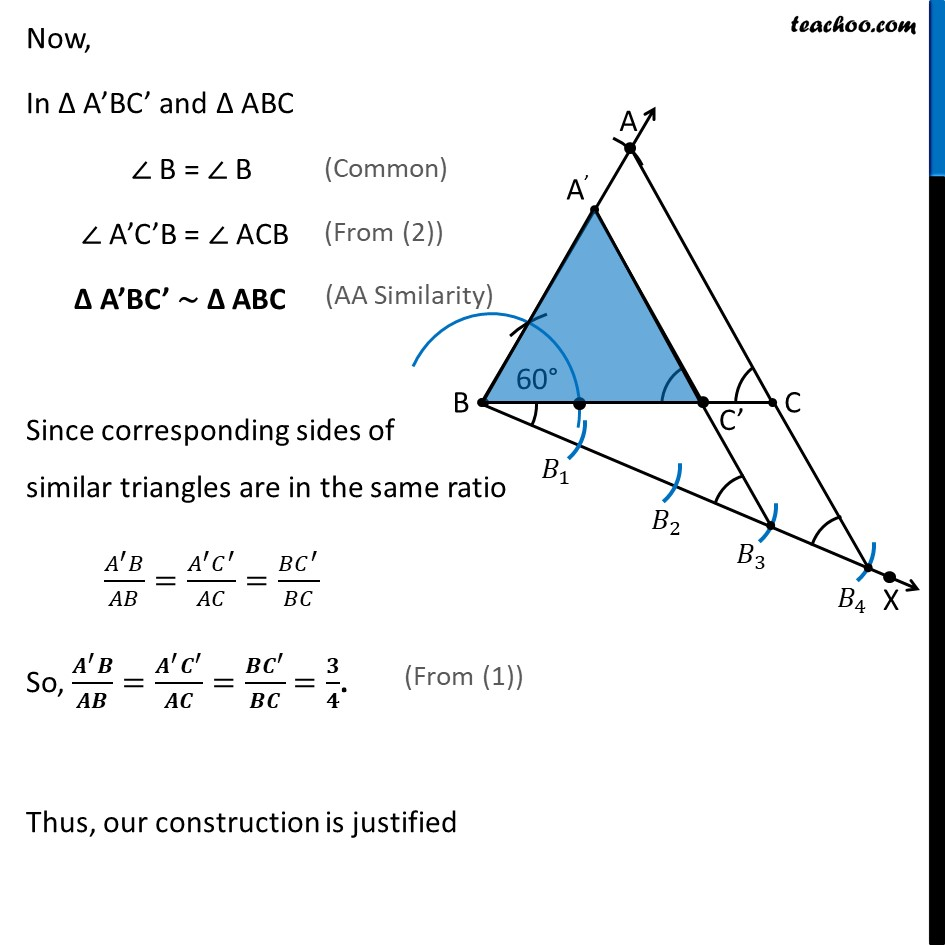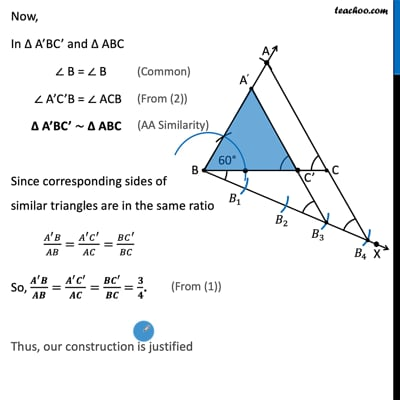This video is only available for Teachoo black users

Introducing your new favourite teacher - Teachoo Black, at only ₹83 per month

### Transcript

Ex 11.1, 5 Draw a triangle ABC with side BC = 6 cm, AB = 5 cm and ∠ ABC = 60°. Then construct a triangle whose sides are 3/4 of the corresponding sides of the triangle ABC. Let’s first draw a rough diagram Now, Let’s construct it Steps to draw Δ ABC Draw base BC of side 6 cm Draw ∠ B = 60° Note: To learn how to draw 60°, check Construction 11.1 of Class 9 3. Taking B as center, 5 cm as radius, we draw an arc Let the point where arc intersects the ray be point A 4. Join AC ∴ Δ ABC is the required triangle Now, we need to make a triangle which is 3/4 times its size ∴ Scale factor = 3/4 < 1 Steps of construction Draw any ray BX making an acute angle with BC on the side opposite to the vertex A. Mark 4 (the greater of 3 and 4 in 3/4 ) points 𝐵_1, 𝐵_2, 𝐵_3,𝐵_4 on BX so that 〖𝐵𝐵〗_1=𝐵_1 𝐵_2=𝐵_2 𝐵_3=𝐵_3 𝐵_4 Join 𝐵_4 𝐶 and draw a line through 𝐵_3 (the 3rd point, 3 being smaller of 3 and 4 in 3/4) parallel to 𝐵_4 𝐶, to intersect BC at C′. 4. Draw a line through C′ parallel to the line AC to intersect BA at A′. Thus, Δ A’BC′ is the required triangle Justification Since scale factor is 3/4, we need to prove (𝑨^′ 𝑩)/𝑨𝑩=(𝑨^′ 𝑪^′)/𝑨𝑪=(𝑩𝑪^′)/𝑩𝑪 =𝟑/𝟒. By construction, BC^′/𝐵𝐶=(𝐵𝐵_3)/(𝐵𝐵_4 )=3/4. Also, A’C’ is parallel to AC So, they will make the same angle with line BC ∴ ∠ A’C’B = ∠ ACB Now, In Δ A’BC’ and Δ ABC ∠ B = ∠ B ∠ A’C’B = ∠ ACB Δ A’BC’ ∼ Δ ABC Since corresponding sides of similar triangles are in the same ratio (𝐴^′ 𝐵)/𝐴𝐵=(𝐴^′ 𝐶^′)/𝐴𝐶=(𝐵𝐶^′)/𝐵𝐶 So, (𝑨^′ 𝑩)/𝑨𝑩=(𝑨^′ 𝑪^′)/𝑨𝑪=(𝑩𝑪^′)/𝑩𝑪 =𝟑/𝟒. Thus, our construction is justified